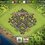# This kinda special limit

Let $P_a$ and $P_b$ be 2 polynomials with same degree, n, and same leading coefficient c, with $c\neq 0$ (otherwise the degree is less than n):

$P_a(x)=cx^n+a_{n-1}x^{n-1}+\displaystyle\sum_{i=0}^{n-2}a_ix^i$

$P_b(x)=cx^n+b_{n-1}x^{n-1}+\displaystyle\sum_{i=0}^{n-2}b_ix^i$

Let $y=\displaystyle\lim_{x\rightarrow\infty}\Big(\sqrt[n]{P_a(x)}-\sqrt[n]{P_b(x)}\Big)$. In this note I try to find the value of $y$.

Let $L_a(x)=\displaystyle\sum_{i=0}^{n-2}a_ix^i$, and $L_b(x)=\displaystyle\sum_{i=0}^{n-2}b_ix^i$. Later, we'll see that both the value of $L_a(x)$ and $L_b(x)$ won't affect the value of the limit. We have:

$y=\displaystyle\lim_{x\rightarrow\infty}\sqrt[n]{c}\Bigg(\sqrt[n]{x^n+\dfrac{a_{n-1}}{c}x^{n-1}+\dfrac{L_a(x)}{c}}-\sqrt[n]{x^n+\dfrac{b_{n-1}}{c}x^{n-1}+\dfrac{L_b(x)}{c}}\Bigg)$

$y=\sqrt[n]{c}\displaystyle\lim_{x\rightarrow\infty}\dfrac{\bigg(x^n+\dfrac{a_{n-1}}{c}x^{n-1}+\dfrac{L_a(x)}{c}\bigg)-\bigg(x^n+\dfrac{b_{n-1}}{c}x^{n-1}+\dfrac{L_b(x)}{c}\bigg)}{\displaystyle\sum_{i=0}^{n-1}\Bigg(\sqrt[n]{\frac{P_a(x)}{c}}\Bigg)^i\Bigg(\sqrt[n]{\frac{P_b(x)}{c}}\Bigg)^{n-1-i}}$

$y=\dfrac{\sqrt[n]{c}}{c}\displaystyle\lim_{x\rightarrow\infty}\dfrac{(a_{n-1}-b_{n-1})x^{n-1}+\big(L_a(x)-L_b(x)\big)}{\displaystyle\sum_{i=0}^{n-1}\Bigg(\sqrt[n]{\dfrac{P_a(x)}{c}}\Bigg)^i\Bigg(\sqrt[n]{\dfrac{P_b(x)}{c}}\Bigg)^{n-1-i}}$

$y=\dfrac{\sqrt[n]{c}}{c}\displaystyle\lim_{x\rightarrow\infty}\dfrac{a_{n-1}-b_{n-1}+\dfrac{L_a(x)-L_b(x)}{x^{n-1}}}{\displaystyle\sum_{i=0}^{n-1}\Bigg(\sqrt[n]{\dfrac{P_a(x)}{cx^n}}\Bigg)^i\Bigg(\sqrt[n]{\dfrac{P_b(x)}{cx^n}}\Bigg)^{n-1-i}}$

Since $\deg(P_a)=\deg(P_b)=n$ and both have leading coefficient c, then:

$y=\dfrac{\sqrt[n]{c}}{c}\displaystyle\lim_{x\rightarrow\infty}\frac{a_{n-1}-b_{n-1}}{\displaystyle\sum_{i=0}^{n-1}1^{i}1^{n-1-i}}$

$y=\dfrac{\sqrt[n]{c}}{c}\dfrac{a_{n-1}-b_{n-1}}{n}$

$y=\dfrac{a_{n-1}-b_{n-1}}{n\sqrt[n]{c^{n-1}}}$

and we're done. We've found the value of $y$. Take quite long, around 1 hour.Note by Gian Sanjaya
5 years, 8 months ago

This discussion board is a place to discuss our Daily Challenges and the math and science related to those challenges. Explanations are more than just a solution — they should explain the steps and thinking strategies that you used to obtain the solution. Comments should further the discussion of math and science.

When posting on Brilliant:

• Use the emojis to react to an explanation, whether you're congratulating a job well done , or just really confused .
• Ask specific questions about the challenge or the steps in somebody's explanation. Well-posed questions can add a lot to the discussion, but posting "I don't understand!" doesn't help anyone.
• Try to contribute something new to the discussion, whether it is an extension, generalization or other idea related to the challenge.
• Stay on topic — we're all here to learn more about math and science, not to hear about your favorite get-rich-quick scheme or current world events.

MarkdownAppears as
*italics* or _italics_ italics
**bold** or __bold__ bold
- bulleted- list
• bulleted
• list
1. numbered2. list
1. numbered
2. list
Note: you must add a full line of space before and after lists for them to show up correctly
paragraph 1paragraph 2

paragraph 1

paragraph 2

[example link](https://brilliant.org)example link
> This is a quote
This is a quote
    # I indented these lines
# 4 spaces, and now they show
# up as a code block.

print "hello world"
# I indented these lines
# 4 spaces, and now they show
# up as a code block.

print "hello world"
MathAppears as
Remember to wrap math in $$ ... $$ or $ ... $ to ensure proper formatting.
2 \times 3 $2 \times 3$
2^{34} $2^{34}$
a_{i-1} $a_{i-1}$
\frac{2}{3} $\frac{2}{3}$
\sqrt{2} $\sqrt{2}$
\sum_{i=1}^3 $\sum_{i=1}^3$
\sin \theta $\sin \theta$
\boxed{123} $\boxed{123}$

## Comments

Sort by:

Top Newest

You should state what you are trying to achieve at the start, so that others can understand what you are trying to say.

Staff - 5 years, 8 months ago

Log in to reply

Oh okay, thanks for the suggestion. I'll edit it.

- 5 years, 8 months ago

Log in to reply

Thanks. This clarifies the result :)

What happens if $\deg P_a = n$ and $\deg P_b = m$? What would be the conditions under which $\lim \sqrt[n]{P_a} - \sqrt[m]{P_b}$ is a constant? And under these conditions, what is the constant equal to?

Staff - 5 years, 8 months ago

Log in to reply

Let the leading coefficient of $P_a$ and $P_b$ be $z_a$ and $z_b$, respectively. I would consider separating the root of polynomials in the limit to get:

$\displaystyle \lim_{x\rightarrow\infty} \Big(\sqrt[n]{P_a(x)} - \sqrt[m]{P_b(x)}\Big)=\displaystyle \lim_{x\rightarrow \infty} \Big(\sqrt[n]{P_a(x)} - \sqrt[n]{z_a}x\Big) - \displaystyle \lim_{x\rightarrow \infty} \Big(\sqrt[m]{P_b(x)} - \sqrt[m]{z_b}x\Big) + \displaystyle \lim_{x\rightarrow\infty} \Big(\sqrt[n]{z_a} - \sqrt[m]{z_b}\Big)x$

Applying what I've found, we would see that the first 2 limits are finite; the whole limit will be constant, not approaching either sides of infinity, if and only if the last limit is constant, which happens if and only if $\sqrt[n]{z_a}-\sqrt[m]{z_b}=0$, or equivalently $\sqrt[n]{z_a}=\sqrt[m]{z_b}$. Now, let $z_a = c^n$ and $z_b=c^m$ for a positive real number c (so it holds for all values of m and n), and let $P_a$ and $P_b$ be defined as:

$P_a(x)=c^n x^n + a_{n-1} x^{n-1} + \displaystyle \sum_{i=0}^{n-2} a_i x^i$

$P_b(x)=c^m x^m + b_{m-1} x^{m-1} + \displaystyle \sum_{i=0}^{m-2} b_i x^i$

We now have:

$\displaystyle \lim_{x\rightarrow\infty} \Big(\sqrt[n]{P_a(x)} - \sqrt[m]{P_b(x)}\Big)=\frac{a_{n-1}}{nc^{n-1}}-\frac{b_{m-1}}{mc^{m-1}}$

- 5 years, 8 months ago

Log in to reply

That's a good analysis.

Pointers
1. Your end result disagrees with the previous result.
2. When you split out a limit (LHS) into 2 or more parts (RHS), it is not true that the LHS exists if and only if the RHS exists. If the RHS exists, then the LHS exists. But it is possible for the LHS to exist, while the RHS does not exist. Case in point:
$\lim 0 \neq \lim x + \lim (-x).$
3. I suggest doing something similar to what you did. Consider $( a^{nm} - b^{nm}) = (a-b) \times$ stuff.

Staff - 5 years, 8 months ago

Log in to reply

×

Problem Loading...

Note Loading...

Set Loading...